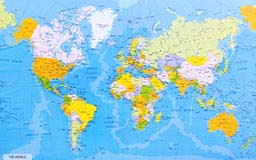# Earth Geography Quiz

0

Earth Geography QuizEarth Geography Quiz

## Earth Geography Quiz

Q: What is the total amount of soil on the Earth?

A. 51,01,00,500 sq. Km.

Q: What is the age of the earth?

Q: What is the equatorial circumference of the earth?

A. North 40,067 km (east-west).

Q: What is the circumference of the Earth’s pole?

A. 40,000 km (north-south).

Q: What is the diameter of the earth’s spinal cord?

A. 12,714 km.

Q: What is the density of the earth?

A. 5.517 (taking water density to be 1).

Q. What is the percentage of the water area of the earth?

A. 70.08 per cent.. Earth Geography Quiz

Q: What is the size of the earth?

A. 1,083,208,840,000 cubic kilometers.

Q. What is the mass of the earth?

A. 5.88 × 1021 tons.

Q. What is the total land area of the earth?

A. 29.92 per cent.

Q: What is the period of rotation of the earth?

A. 365 days 6 hours 9 minutes 10 seconds.

Q: What is the average distance of the earth from the sun?

A. 14.96 crore kilometres.

Q: What is the speed of the Earth’s orbit?

A. 29.8 kilometres per second.

Q: What is the period of rotation of the earth?

A. 23 hours 56 minutes 4.01 seconds.

Q: What is the angle between the earth and the sun?

A. 23 degrees.

Q: What is the angle of the Earth’s spine with the orbit?

A. 66 degrees.

Q: How many continents are there on the Earth?

A. 7 .

Q. How many oceans are there on the Earth?

A. 5 . Earth Geography Quiz

Q. What is the total number of countries in the world?

A. 196 (as per 2013 census).

Q: How long does it take for the sun’s rays to reach the earth’s surface?

A. 8 minutes 18 seconds.

Q: What is the distance of the moon from the earth?

A. 3,84,400 km.

Q: How many satellites are there of the Earth?

A. 1.. Earth Geography Quiz

Q: What is the name of the Earth’s satellite?

A. The moon.

Q: What are the two motions of the Earth?

A. Earth’s motion is two – diurnal motion and annual motion.

Q. Which is the longest day of the year?

A. June 21.

Q: Which is the shortest day of the year?

A. 22 December.

Q. Which day of the year is equal to day and night?

A. March 21 and September 23.

Q. Which is the largest continent in the world?

A. Asia. Earth Geography Quiz

Q: Which is the smallest country in the world?

A. Vatican City.

Q: Which is the smallest continent in the world?

A. Australia.

Q: Which is the most recent country in the world?

A. South Chudan (2011).  *0 0 0*.

Earth Geography Quiz

You May Like:

Previous article Assam History Quiz
Next articleIndia Geography Quiz